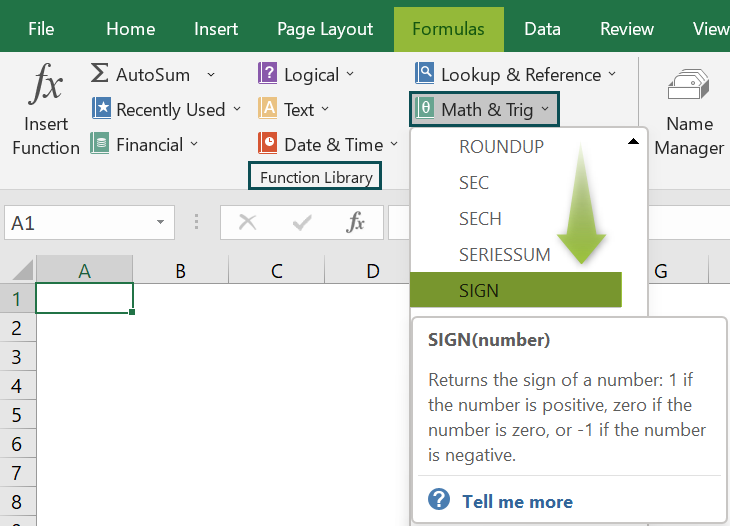# SIGN Function in ExcelArticle byExcelMojo Team## What Is SIGN Function In Excel?

The SIGN Excel function returns the preset return values based on the SIGN of the real integers or the numeric values in a given dataset. The return values are ‘-1’ for a negative number, ‘0’ for a 0 cell value, and ‘+1’ for a positive number. We can get the values directly or find the SIGN for the results of a formula.

The SIGN function in Excel is an inbuilt “Maths & Trig” function, so we can insert the formula from the “Function Library” or enter it directly in the worksheet.

For example, we will use the SIGN function to find the SIGN for the given data.

Select cell B2, enter the formula =SIGN(A2-A3), and press the “Enter” key.

The result is returned as “-1”, as shown above.

[Output Observation: In cell B2, first, the difference of the cells is calculated, (A2-A3), i.e., (4-7), and the result is -3. Since the result is a negative number, the return value of the SIGN is -1, as shown.]

###### Key Takeaways
• The SIGN Excel function checks the SIGN of the entered number, whether it is positive, 0, or a negative number, and returns the respective value.
• The result can be the direct SIGN value of the selected numeric value, or the SIGN value of the result of an executed formula.
• Also, the function returns the SIGN values as follows:
• If the “number” is in the range “-∞, 0”, it will return -1.
• If the “number” is equal to “0”, it will return 0.
• If the “number” is in the range “0, ∞”, it will return 1.

### SIGN() Excel Formula

The syntax of the SIGN Excel formula is,

The argument of the SIGN Excel formula is,

• number: It is a mandatory argument. It is a real number or integer value to find the SIGN value.

### How To Use SIGN Excel Function?

We can use the SIGN Excel function in 2 ways, namely,

1. Access from the Excel ribbon.
2. Enter in the worksheet manually.

#### Method #1 – Access from the Excel ribbon

Choose an empty cell for the output → select the “Formulas” tab → go to the “Function Library” group → click the “Math & Trig” option drop-down → select the “SIGN” function, as shown below.

The “Function Arguments” window appears. Enter the argument in the “Number” field → click “OK”, as shown below.

#### Method #2 – Enter in the worksheet manually

1. Select an empty cell for the output.
2. Type =SIGN( in the selected cell. [Alternatively, type =S or =SI and double-click the SIGN function from the list of suggestions shown by Excel.]
3. Enter the argument as cell value or excel cell reference and close the brackets.
4. Press the “Enter” key.

Let us take an example to learn its usage.

We will find the positive SIGN of the values using SIGN Excel function.

In the table, the data is,

• Column A contains the Value1.
• Here, column B contains the Value2.
• Column C displays the Output.

The steps to calculate the value using SIGN formula are,

1. Select cell C2, enter the formula =SIGN(A2-B2), and press the “Enter” key. The result is “1”, as the difference between cells A2 and B2 is a positive number.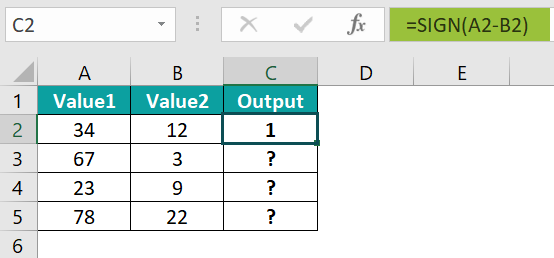2. Drag the formula from cell C2 to C5 using the fill handle.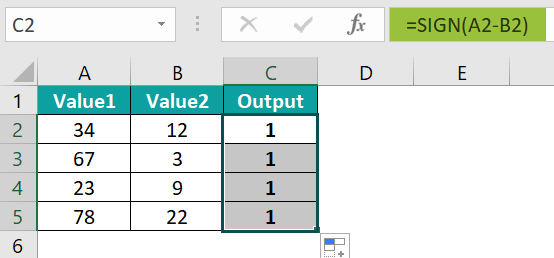The output is shown above as +1 since the difference of the values are all positive numbers.

### Examples

We will understand some advanced scenarios using the SIGN Excel function examples.

#### Example #1

We will find the negative SIGN of the values using the SIGN function.

In the table, the data is,

• Column A contains the Value1.
• Here, column B contains the Value2.
• Column C displays the Output.

The steps to calculate the value by the SIGN formula are,

• Step 1: Select cell C2, enter the formula =SIGN(A2-B2), and press the “Enter” key. The result is “-1”, as the difference between cells A2 and B2 is a negative number.

The output is shown above as -1 since the difference of the values are all negative numbers.

#### Example #2

We will calculate the zero SIGN of the values using the SIGN function.

In the table, the data is,

• Column A contains the Value1.
• Here, column B contains the Value2.
• Column C displays the Output.

The steps to calculate the value by the SIGN formula are,

• Step 1: Select cell C2, enter the formula =SIGN(A2-B2), and press the “Enter” key. The result is “0”, as the difference between cells A2 and B2 is 0.
• Step 2: Drag the formula from cell C2 to C5 using the fill handle.

The output is shown above as 0 since the difference of the values are all 0.

#### Example #3

We will calculate the errors using the SIGN function.

In the table, the data is,

• Column A contains the Value1.
• Here, column B contains the Value2.
• Column C displays the Output.

The steps to calculate the value by the SIGN formula are,

• Step 1: Select cell C2, enter the formula =SIGN(A2-B2), and press the “Enter” key. The result is a “#VALUE!” error, as the difference between cells A2 and B2 is an error.
• Step 2: Drag the formula from cell C2 to C5 using the fill handle.

The output is shown above as errors since the difference of the values are all errors as the dataset consists of textual values, symbols, etc.

### Important Things To Note

• The “#VALUE!” error occurs when the argument entered contains a non-numeric value.
• When the cell value is a text directly entered as an argument, we get the “#NAME?” error.
• We get the “#SPILL!” error when we choose a cell range for the number argument. Therefore, we must select only one cell reference for the function and not a cell range.

1. What is the SIGN Excel function used for?

The SIGN Excel function specifies that the number entered is either negative, positive, or equal to 0.

The syntax of the SIGN function is =SIGN(number)

2. How to insert the SIGN Excel function?

To insert the SIGN function in Excel, follow the following steps;

1. Select an empty cell for the output.
2. Type =SIGN( in the selected cell. [Alternatively, type =S or =SI and double-click the SIGN function from the list of suggestions shown by Excel.]
3. Enter the argument as cell value or cell reference and close the brackets.
4. Press the “Enter” key.

For example, we will calculate the SIGNs using the SIGN formula for the values in different formats.

In the table, the data is,
Column A contains the Value.
Column B displays the Output.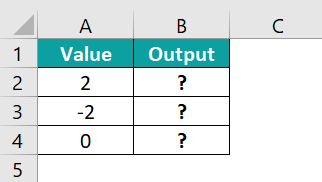The steps to calculate the value by the SIGN formula are as follows:

• Step 1: Select cell B2, enter the formula =SIGN(A2), and press the “Enter” key. The result is “1”, as shown below.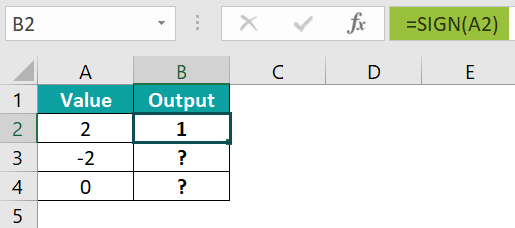• Step 2: Drag the formula from cell B2 to B4 using the fill handle.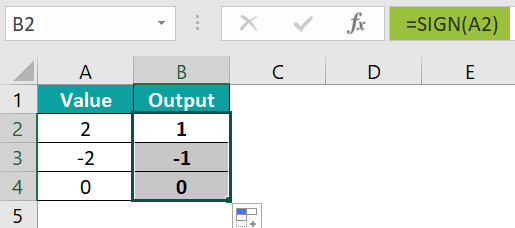Therefore, the SIGN function shows the following results.
Positive, such as 2, the function returns a 1.
Negative, such as -2, the function returns a -1.
Zero, the function returns a 0.

3. Where is the Excel SIGN function?

The Excel SIGN Function is in the “Formula” tab and is inserted as follows:

Choose an empty cell for the output → select the “Formulas” tab → go to the “Function Library” group → click the “Math & Trig” option drop-down → select the “SIGN” function, as shown below.